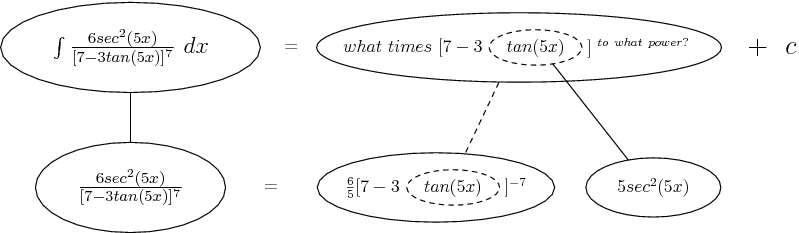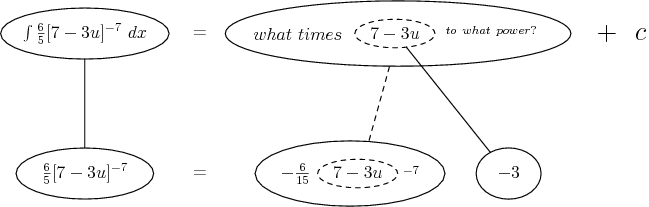# Thread: solve the question of calculas

1. ##solve the question of calculas

kindly solve the question which i am writing down:

Evaluate the following definite integral using the substitution method:

6sec^2(5x) dx
[7-3tan(5x)]^7

2.Originally Posted by hira fareedkindly solve the question which i am writing down:

Evaluate the following definite integral using the substitution method:

6sec^2(5x) dx
[7-3tan(5x)]^7
I don't do solve.

Here's a push in the right direction: Substitute $\displaystyle u = \tan (5x) \Rightarrow dx = \frac{du}{5 \sec^2 (5x)}$.

3. And, just in case a picture helps...... where straight continuous lines differentiate (downward) or integrate (upward) with respect to x, and the straight dashed line with respect to the dashed balloon expression. So that the right-hand, triangular network satisfies the chain-rule for differentiation. To fill in the blanks, you need to do the 'dashed' integration, as though the dashed balloon were just a variable like x or u. But actually this too involves the chain rule, so you might like to considerAnd now you can complete both pictures by doing $\displaystyle \int-\frac{6}{15}t^{-7}dt$

Don't integrate - balloontegrate! Balloon Calculus: worked examples from past papers

#### Search Tags

calculas, hira fareed, question, solve